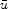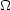### IV. PARTICLE CANDIDATES

Although the existence of dark matter is well motivated by several lines of evidence, the exact nature of dark matter remains elusive. Dark matter candidates are generically referred to as WIMPs (Weakly Interacting Massive Particles); in other words, they are massive particles that are electrically neutral which do not interact very strongly with other matter. In this section we will explore some possible particle candidates for dark matter and the theories that lie behind them. But to begin with, we give a brief review of the Standard Model of particle physics.

The Standard Model (SM) is the quantum field theory that describes three of the four fundamental forces in nature: electricity and magnetism, the weak nuclear force, and the strong nuclear force. Gravitational interactions are not part of the SM; at energies below the Planck scale gravity is unimportant at the atomic level. There are sixteen confirmed particles in the SM, seven of which were predicted by the model before they were found experimentally, and one particle yet to be seen: the Higgs boson, which is believed to be the mediator of the Higgs field responsible for giving all other SM particles mass. In the SM, there are six quarks (up, down, top, bottom, charm, and strange), six leptons (electron, mu, tau, and their respective neutrinos), and five force carriers (photons, gluons, W±, Z, and the Higgs boson). Quarks and leptons are classified as fermions with half integer spins and are split into three generations, where force carriers are classified as gauge bosons with integer spins. Each of these particles also has a corresponding antiparticle, denoted with a bar (for example, the up antiquark's symbol is), with opposite charge. Table I arranges the SM fundamental particles and some of their basic qualities.

 Generation 1 Generation 2 Generation 3 Particle Mass (MeV) Charge Particle Mass (MeV) Charge Particle Mass (MeV) Charge up quark (u) 2.55 +2/3 charm quark (c) 1270 +2/3 top quark (t) 171200 +2/3 down quark (d) 5.04 -1/3 strange quark (s) 104 -1/3 bottom quark (b) 4200 -1/3 electron (e-) 0.511 -1 muon (µ-) 105.7 -1 tau (-) 1776.8 -1 e neutrino (ve) < 2.0 × 10-6 0 µ neutrino (vµ) <0.19 0neutrino (v) < 18.2 0

 Particle Force Acts through Acts on Mass (MeV) Charge Photon () Electromagnetic Electric charge Electrically charged particles < 1× 10-240 0 Z boson (Z) Weak nuclear Weak interaction Quarks and leptons 91188 0 W± bosons (W±) Weak nuclear Weak interaction Quarks and leptons 80398 ±1 Gluon (g) Strong nuclear Color charge Quarks and gluons 0 0 Higgs boson (H0) Higgs force Higgs field Massive particles > 114400 0

Table I: The particles predicted by the Standard Model. Approximate masses of particles as last reported by the Particle Data Group. 

SM particle interactions obey typical conservation of momentum and energy laws as well as conservation laws for internal gauge symmetries like conservation of charge, lepton number, etc. The model has been thoroughly probed up to energies of1 TeV, and has led to spectacular results such as the precision measurement of the anomalous magnetic moment of the electron (analogous to measuring the distance between New York and Los Angeles to the width of a human hair).

The final undiscovered particle, the Higgs boson, is thought to be extremely massive; the latest bounds from the CDF and DØ collaborations at the Tevatron have restricted the mass of the Higgs to two regions: 114-160 GeV and 170-185 Gev.  Since the Higgs boson couples very weakly to ordinary matter it is difficult to create in particle accelerators. Hopefully, the powerful Linear Hadron Collider (LHC) in Geneva, Switzerland, will confirm the existence of the Higgs boson, the final particle of the SM.

Despite its success, the SM does not contain any particle that could act as the dark matter. The only stable, electrically neutral, and weakly interacting particles in the SM are the neutrinos. Can the neutrinos be the missing dark matter? Despite having the "undisputed virtue of being known to exist" (as put so well by Lars Bergstrom), there are two major reasons why neutrinos cannot account for all of the universe's dark matter. First, because neutrinos are relativistic, a neutrino-dominated universe would have inhibited structure formation and caused a "top-down" formation (larger structures forming first, eventually condensing and fragmenting to those we see today).  However, galaxies have been observed to exist less than a billion years after the big bang and, together with structure formation simulations, a "bottom-up" formation (stars then galaxies then clusters etc.) seems to be the most likely.  Second, Spergel et al. ruled out neutrinos as the entire solution to missing mass using cosmological observations: WMAP combined with large-scale structure data constrains the neutrino mass to mv < 0.23 eV, which in turn makes the cosmological densityv h2 < 0.0072.  While neutrinos do account for a small fraction of dark matter, they clearly cannot be the only source.

The lack of a dark matter candidate does not invalidate the SM, but rather suggests that it must be extended. Perhaps the SM is only a valid theory at low energies, and that there is new physics "beyond the Standard Model;" that is, new theories may supplement, rather than replace, the SM. Such new theories have already been proposed, the most promising being supersymmetry, which also yields a viable dark matter candidate called the neutralino or LSP.

Although very successful, the SM has two flaws which hint at the need for new solutions: the hierarchy problem and the fine-tuning problem. The hierarchy problem arises from the SM's prediction of the Higgs vacuum expectation value (vev), which is about 246 GeV. Theorists have predicted that at high enough energies (1 TeV) the electromagnetic and weak forces act as a single unified force called the electroweak force (this has also been experimentally verified). However at smaller energies, the single unified force breaks down into two separate forces: the electromagnetic force and the weak force. It turns out that after this breaking, the Higgs field's lowest energy state is not zero, but the 246 GeV vacuum expectation value. It is precisely this non-zero value that gives other particles mass through their interactions with the Higgs field. The 246 GeV vev is at the weak scale (the typical energy of electroweak processes); however, the Planck scale (the energy at which quantum effects of gravity become strong) is around 1019 GeV. The basic question, then, is why is the Planck scale 1016 times larger than the weak scale? Is there simply a "desert" between 103 and 1019 GeV in which no new physics enters?

There is an additional difficulty with the Standard Model. Most calculations in a quantum field theory are done perturbatively. For example, the scattering cross section of two electrons at a given energy can be calculated up to a certain power of, the fine structure constant, which is the coupling constant for electromagnetism. The calculation is represented pictorially with Feynman diagrams; the number of particle interaction vertices is related to the power of. However, virtual particles and more complicated diagrams can also contribute to the process with higher powers of.Figure 4. The tree level diagram on the left represents the electron-electron scattering process to the lower order in perturbation theory. The two graphs on the right represent higher order processes (which can be thought of as loop corrections) and enter with higher powers of.

As an example, the best anomalous magnetic moment of the electron calculation involves 891 diagrams.  Thus most quantities have so-called "quantum-loop" corrections (although some quantities, like the photon's mass, are protected by symmetries). The fine-tuning problem arises when trying to calculate the mass of the Higgs particle; quantum loop corrections to the Higgs mass are quadratically divergent. If one uses 1016 GeV as the scale at which the electroweak and strong forces combine to become a single unified force (which has been theorized but not seen), one requires an almost perfect cancellation on the order of 1 part in 1014 for the Higgs mass to come out at the electroweak scale (150 GeV). This unnatural cancellation is a source of alarm for theorists and signifies that we lack an understanding of physics beyond the SM.